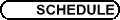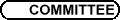SS9 - Analyse variationnelle et optimisation / SS9 - Variational analysis and optimization
Org: J-B Hiriart-Urruty (Toulouse) et/and A. Lewis (Simon Fraser)

CHARLES AUDET, Ecole Polytechnique de Montréal
Mesh Adaptive Direct Search algorithms for constrained optimization

We introduce the Mesh Adaptive Direct Search (MADS) class of algorithms for nonlinear optimization. MADS extends the Generalized Pattern Search (GPS) class by allowing local exploration, called polling, in a dense set of directions in the space of optimization variables. This means that under certain hypotheses, including a weak constraint qualification due to Rockafellar, MADS can treat constraints by the extreme barrier approach of setting the objective to infinity for infeasible points and treating the problem as unconstrained. The main GPS convergence result is to identify limit points where the Clarke generalized derivatives are nonnegative in a finite set of directions, called refining directions. Although in the unconstrained case, nonnegative combinations of these directions spans the whole space, the fact that there can only be finitely many GPS refining directions limits rigorous justification of the barrier approach to finitely many constraints for GPS. The MADS class of algorithms extend this result; the set of refining directions may even be dense in Rn.

We present an implementable instance of MADS, and we illustrate and compare it with GPS on some test problems.

This is joint work with John Dennis.

HEINZ BAUSCHKE, University of Guelph, Ontario, Canada
Minimization with alternating Bregman proximity operators

We investigate two notions of proximity operators associated with Bregman distances. These operators are shown to inherit key properties of the standard Moreau proximity operator. Through this analysis, we establish the convergence of an alternating minimization procedure for solving a class of optimization problems.

Based on joint work with Patrick Combettes (Paris 6) and Dominik Noll (Toulouse).

J. FRÉDÉRIC BONNANS, INRIA-Rocquencourt
Optimal control of elliptic variational inequalities

In a recent work with R. Bessi Fourati and H. Smaoui we discussed a variant of the standard obstacle problem in which forces derive from a given amount of liquid, above of the membrane; the position of the liquid is itself an unknown of the problem. Here we consider associated optimal control problems through an external force field, or through the obstacle itself. There are two main cases. If the domain is small enough then the state (vertical deformation of membrane) is a well-defined function of control; otherwise the system may be ill-posed. In both cases, regularization schemes allow to obtain optimality systems.

This is a joint work with R. Bessi Fourati, Univ. Sousse (Tunisia).

## References


R. Bessi Fourati, J. F. Bonnans and H. Smaoui, The obstacle problem for water tanks. J. Math. Pures Appl. 82(2003), 1527-1553.

PATRICK-LOUIS COMBETTES, Université Paris 6
Finding zeros of co-hypomonotone operators

Conditions are given for the viability and the weak convergence of an inexact, relaxed proximal point algorithm for finding a common zero of countably many cohypomonotone operators in a Hilbert space. In turn, new convergence results are obtained for an extended version of the proximal method of multipliers in nonlinear programming.

Joint work with T. Pennanen.

JEAN-NOËL CORVELLEC, Université de Perpignan, 52 avenue Paul Alduy, 66860 Perpignan Cedex
On nonlinear error bounds

Dans la continuité de nos travaux récents avec D. Azé sur la caractérisation des bornes d'erreur pour les fonctions semi-continues inférieurement définies sur un espace métrique complet (le cas "linéaire"), nous présentons des extensions (partielles) au cas non linéaire, et quelques-unes de leurs conséquences en relation avec des résultats récents de la littérature.

Following our recent work with D. Azé about the characterization of error bounds for lower semicontinuous functions defined on complete metric spaces (the "linear case"), we present some (partial) extensions in the nonlinear case, as well as some of their consequences related to various recent results in the literature.

ROBERT DEVILLE, Université Bordeaux 1, 351 cours de la Libération, 33400 Talence
Ensemble des dérivées d'une application différentiable

Nous présentons les résultats obtenus récemment sur la structure de l'ensemble des dérivées d'une application différentiable entre deux espaces de Banach X et Y. Les résultats dépendent de la géometrie de X et Y, de la notion de différentiabilité utilisée, et de la structure globale de l'application considerée. Nous présentons en particulier des exemples d'applications Lipschitziennes de X dans Y, bornées, Gateaux-différentiables en tout point, telles que pour tout x, y dans l'espace de départ X, la norme de f¢(x)-f¢(y) est plus grande que 1. Une telle construction peut être faite lorsque X et Y sont des espaces de Hilbert séparables de dimension infinie.

JEAN-CHARLES GILBERT, INRIA Rocquencourt
On the solution of convex quadratic optimization problems by augmented Lagrangian and active set methods

We discuss the use of an augmented-Lagrangian-active-set algorithm for solving convex quadratic optimization problems. A global linear convergence is shown when the augmentation parameter is larger than a certain (usually unknown) Lipschitz constant and the inner subproblems are solved exactly. The result follows from a lemma of general interest dealing with the projection onto a convex polyhedron. The case when the inner subproblems are solved approximately is also considered. This convergence result leads to a discussion on the iterative complexity of such algorithms.

On the other hand, it is argued that an augmented-Lagrangian-active-set algorithm is appropriate to solve the QP's arising from the use of an SQP-Gauss-Newton method to solve constrained nonlinear least-squares problems. Numerical experiments in the seismic tomography domain are given as an illustration.

JEAN-LOUIS GOFFIN, Faculty of Management, McGill University, 1001 Sherbrooke Street West, Montréal, Québec H3A 1G5
Conic column generation

Dantzig-Wolfe column generation can be formulated in the case where columns belong to a general self-dual cone. By duality this leads to a variant of the cutting plane method where the cutting planes may be linear, SOCC or SDP, thus leading to approximating an NDO function by SOCC or SDP (i.e. nonlinear or non-differentiable) cuts. Complexity as well as computational results will be presented.

DOMINIKUS NOLL, Université Paul Sabatier
Optimization methods for feedback control

Optimization for feedback control is a recent and very active field with many challenging problems and a large potential for applications ahead. Semidefinite programming (SDP) is one of those techniques which come to mind as having led to significant progress over the last decade in solving problems in control, finance and integer programming relaxations. However, despite these merits, the use of SDP for feedback control design is limited since most problems of practical interest are non-convex and require different algorithmic approaches. In this talk we shall discuss two recent strategies, programming under bilinear matrix inequality (BMI) constraints, and nonsmooth methods based on bundling techniques. These approaches are suited for many of the challenging NP-hard problems in controller synthesis.

HRISTO SENDOV, University of Guelph
Differentiating Spectral Functions

A function F on the space of n ×n symmetric matrices is called spectral if it depends only on the eigenvalues of its argument, that is F(A) = F(UAUT) for every orthogonal U and every A in its domain. We are interested in the derivatives of these functions with respect to the argument A. Explicit formulae for the gradient and the Hessian are given in , . These formulae appear quite different from each other and this obstructs attempts to generalize them. The questions that we address in this talk are about the common features in these formulae. We propose a language that seems to describe well the higher-order derivatives of the spectral functions. It is based on a generalization of the Hadamard product between two matrices to a (tensor-valued) product between k matrices, for k ³ 1.

In particular, we present a compact formula for the k-th derivative of the operator-valued function f(A), where f is a function on a scalar argument, see [3,Chapter V].

## References


A. S. Lewis, Derivatives of spectral functions. Math. Oper. Res. 21(1996), 576-588.


A. S. Lewis and H. S. Sendov, Twice Differentiable Spectral Functions. SIAM J. Matrix Anal. Appl. (2) 23(2001), 368-386.


R. Bhatia, Matrix Analysis. Springer-Verlag, NY, 1997.

MICHEL THERA, LACO, Université de Limoges
A converse of the Eidelheit theorem in real Hilbert spaces

This talk is based on a recent work with E. Ernst in which we establish the following converse of the Eidelheit theorem: an unbounded closed and convex set of a real Hilbert space may be separated by a closed hyperplane from every other disjoint closed and convex set, if and only if it has a finite codimension and a nonempty interior with respect to its affine hull.

LIONEL THIBAULT, Université Montpellier 2
On saddle functions in Banach spaces

The talk will be focused on some important questions related to the analysis of extended real valued saddle functions defined on the product of two real Banach spaces. I will present some new results obtained recenty with N. Zlateva.